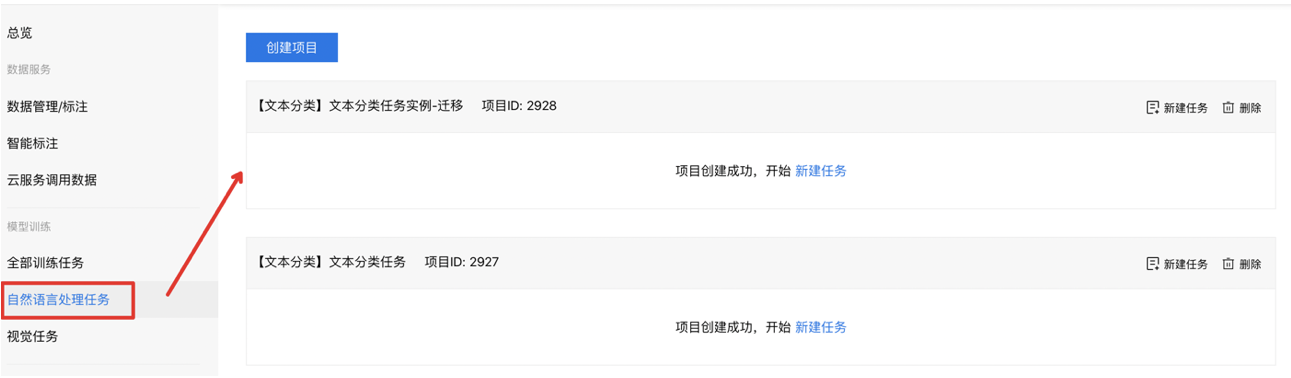# 代码模板升级及迁移说明

## 一、代码模板升级整体说明

平台于2020年4月16日，对自然语言处理模型训练的代码模板进行了升级，建议您在5月1日前完成对旧版任务的迁移。本次升级，平台的自然语言处理任务将为您提供封装性和灵活性俱佳的开发体验，并支持了飞桨深度学习框架Paddle-Fluid 1.6.1的版本。

针对平台升级新版本模型代码框架，请您关注如下几个时间点：

1. 平台于4月16日，更新上线新版代码框架，暂时保留对旧版任务的克隆功能
2. 平台于5月1日，将不再提供旧版本代码模板的克隆。当您对旧版代码模板克隆任务时，平台仅提供新版代码框架，请您做好代码的迁移工作。

说明：您在5月1日前，基于旧版本代码模板发布的任务，可长期在线上进行云端调用或本地使用。

后续平台也将基于升级后框架，继续新增任务类型，您可以加入BML用户交流群，针对您在模型开发过程中的需要，提出您所需的功能需求。

## 二、如何操作迁移到新版任务下

将老版本任务迁移到新版本的任务，以分类任务为例可分为三步：

第一步：查看老版本的任务中，代码编辑部分，存在哪些字段的修改

在任务列表中，找到对应的任务详情：在代码配置详情中，找到脚本内容部分，查看修改的代码内容：第二步：在平台「自然语言处理」任务列表下，新建项目并新建任务，此任务将会是基于最新的代码模板创建第三部：在下图中代码编辑部分，将第一步中对应修改过的代码部分，在新任务中进行逐一调整（关于新版代码模板的说明，详见下文）## 三、新老版本的代码编辑模板区别说明

您可以通过了解旧版本的代码模板和新版本的代码模板的代码结构，来了解两版代码的编写逻辑，从而方便您将旧版本的代码迁移到新版本的代码模板中。我们将以文本分类任务中，使用ERNIE和BOW网络的设计进行讲解说明。

下面我们先看一下旧版本的代码编辑模板的内容：

### 3.1 旧版代码模板说明

#### 1. 模型训练的配置信息

关于模型训练过程中，关于学习率、batch size等配置，代码如下：

``````def __init__(self):
Config.__init__(self)
self.batch_size = 8  # batch_size大小,尽量设置成2的整数倍
self.learning_rate = 2e-5  # 学习率设置
self.save_steps = 500  # 模型保存的间隔，即训练多少个batch之后保存一次模型，请设置成100的倍数
self.epoch = 3  # 训练多少轮
self.skip_steps = 10  # 间隔多少个batch时打印训练日志``````

组网部分，包括了ERNIE表示、BOW模型表示和分类任务几个部分，代码介绍如下：

#### 2. 网络设计中ERNIE表示的代码部分

`` emb = fluid.layers.sequence_unpad(ernie_output["sequence_output"], length=ernie_input["seq_lens"])``

#### 3. 网络设计中BOW的模型表示代码部分

``````  # bow layer
bow = fluid.layers.sequence_pool(input=emb, pool_type='sum')
bow_tanh = fluid.layers.tanh(bow)
# full connect layer
fc_1 = fluid.layers.fc(input=bow_tanh, size=hid_dim, act="tanh")
fc_2 = fluid.layers.fc(input=fc_1, size=hid_dim2, act="tanh")``````

#### 4. 网络设计中分类器的代码部分

`` logits = fluid.layers.fc(input=fc_2, size=cfg.num_labels)``

#### 5. 网络设计中预测部分的内容

`````` if is_inference:
probs = fluid.layers.softmax(logits)
feed_targets_name = [
ernie_input["src_ids"].name, ernie_input["sent_ids"].name,
ernie_input["pos_ids"].name, ernie_input["input_mask"].name,
ernie_input["seq_lens"].name, ernie_input["task_ids"].name
]
return feed_targets_name, probs``````

#### 6. 网络设计中评估部分的代码

``````ce_loss, probs = fluid.layers.softmax_with_cross_entropy(
logits=logits, label=label, return_softmax=True)
loss = fluid.layers.mean(x=ce_loss)
graph_vars = {
"loss": loss,
"classify_infer": probs,
"label": label
}``````

### 3.2 新版代码模板说明

下文中，我们将介绍新升级的代码模板结构，您可以对比新旧版本对应的结构来进行代码的迁移：

#### 1. 新版本代码结构：模型训练的配置信息

``````TrainerConfig = {
"batch_size": 8,
"learning_rate": 2e-05,
"epoch": 3,
"train_log_step": 10,
"save_model_step": 500
}``````

#### 2. 新版本代码结构：数据读入的部分

`````` fields_dict = self.fields_process(fields_dict, phase)
instance_text_a = fields_dict["text_a"]
record_id_text_a = instance_text_a[InstanceName.RECORD_ID]
text_a_src = record_id_text_a[InstanceName.SRC_IDS]
text_a_pos = record_id_text_a[InstanceName.POS_IDS]
text_a_sent = record_id_text_a[InstanceName.SENTENCE_IDS]
text_a_mask = record_id_text_a[InstanceName.MASK_IDS]
text_a_task = record_id_text_a[InstanceName.TASK_IDS]
text_a_lens = record_id_text_a[InstanceName.SEQ_LENS]
instance_label = fields_dict["label"]
record_id_label = instance_label[InstanceName.RECORD_ID]
label = record_id_label[InstanceName.SRC_IDS]``````

#### 3. 新版本代码结构：读取ERNIE表示

``````
emb_dict = self.make_embedding(fields_dict, phase)
emb = emb_dict["text_a"]
text_a_emb = fluid.layers.sequence_unpad(emb, length=text_a_lens)
``````

#### 4. 新版本代码：模型超参数的设置

``````        hid_dim = 128
hid_dim2 = 96
num_labels = 2``````

#### 5. 新版本代码：BOW模型表示部分

``````   # bow layer
bow = fluid.layers.sequence_pool(input=text_a_emb, pool_type='sum')
bow_tanh = fluid.layers.tanh(bow)``````

#### 6. 新版本代码：分类器部分的代码

``````        fc_1 = fluid.layers.fc(input=bow_tanh, size=hid_dim, act="tanh")
fc_2 = fluid.layers.fc(input=fc_1, size=hid_dim2, act="tanh")
logits = fluid.layers.fc(input=fc_2, size=num_labels)``````

#### 7. 新版本代码：预测部分的内容

``````if phase == InstanceName.SAVE_INFERENCE:
"""保存模型时需要的入参：表示预测时最终输出的结果"""
probs = fluid.layers.softmax(logits)
target_predict_list = [probs]
target_feed_name_list = [text_a_src.name, text_a_pos.name, text_a_sent.name,
text_a_mask.name, text_a_lens.name]
emb_params = self.model_params.get("embedding")
ernie_config = ErnieConfig(emb_params.get("config_path"))
if ernie_config.get('use_task_id', False):
target_feed_name_list.append(text_a_task.name)

forward_return_dict = {
InstanceName.TARGET_FEED_NAMES: target_feed_name_list,
InstanceName.TARGET_PREDICTS: target_predict_list
}
return forward_return_dict``````

#### 8. 新版本代码：loss部分的设置

``````   cost, probs = fluid.layers.softmax_with_cross_entropy(
logits=logits, label=label, return_softmax=True)
avg_cost = fluid.layers.mean(x=cost)
"""PREDICT_RESULT,LABEL,LOSS 是关键字，必须要赋值并返回"""``````上一篇
查看训练结果
下一篇
开发表格预测模型